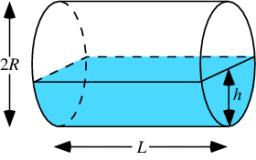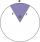# Cylinder horizontally

The cylinder with a diameter of 3 m and a height/length of 15 m is laid horizontally. Water is poured into it, reaching a height of 60 cm below the axis of the cylinder. How many hectoliters of water is in the cylinder?

Correct result:

V =  267.5273 hl

#### Solution:We would be pleased if you find an error in the word problem, spelling mistakes, or inaccuracies and send it to us. Thank you!Tips to related online calculators
Do you want to convert length units?
Do you know the volume and unit volume, and want to convert volume units?

#### You need to know the following knowledge to solve this word math problem:

We encourage you to watch this tutorial video on this math problem:

## Next similar math problems:

• Circular poolThe base of the pool is a circle with a radius r = 10 m, excluding a circular segment that determines the chord length 10 meters. The pool depth is h = 2m. How many hectoliters of water can fit into the pool?
• Horizontal Cylindrical SegmentHow much fuel is in the tank of horizontal cylindrical segment with a length 10m, width of level 1 meter and level is 0.2 meters below the upper side of the tank?
• HectolitersHow many hectoliters of water is in garden barrel with 90 cm diameter and a height of 1.3 m, if it is filled to 80% of its capacity?
• Cylindrical tank9.6 hl of water is poured into a cylindrical tank with a bottom diameter of 1.2 m. What height in centimeters does the water reach?
• Tank and waterCylindrical tank were poured with 3.5 liters of water. If tank base diameter is 3 dm, how height is water level in?
• Circular segmentCalculate the area S of the circular segment and the length of the circular arc l. The height of the circular segment is 2 cm and the angle α = 60°. Help formula: S = 1/2 r2. (Β-sinβ)
• Collect rain waterThe garden water tank has a cylindrical shape with a diameter of 80 cm and a height of 12 dm. How many liters of water will fit into the tank?
• Circular sectorI have a circular sector with a length 15 cm with an unknown central angle. It is inscribed by a circle with radius 5 cm. What is the central angle alpha in the circular sector?
• ConvaHow many liters of water fit into the shape of a cylinder with a bottom diameter 20 cm and a height 45 cm?
• Water tankThe water tank has a cylindrical shape with a base diameter of 4.2 m and is 80 cm deep. How many minutes will take fill it 10 cm below the edge of the tank if water flowing 2 liters per second?
• Maximum of volumeThe shell of the cone is formed by winding a circular section with a radius of 1. For what central angle of a given circular section will the volume of the resulting cone be maximum?
• The potDiameter of the pot 38 cm. The height is 30 cm. How many liters of water can fit in the pot?
• The hemisphereThe hemisphere container is filled with water. What is the radius of the container when 10 liters of water pour from it when tilted 30 degrees?
• ConeCalculate volume and surface area of ​​the cone with a diameter of the base d = 15 cm and side of cone with the base has angle 52°.
• Sphere in coneA sphere of radius 3 cm desribe cone with minimum volume. Determine cone dimensions.
• Prism diagonalThe body diagonal of a regular square prism has an angle of 60 degrees with the base, the edge length is 10 cm. What is the volume of the prism?
• AquariumThe box-shaped aquarium is 40 cm high; the bottom has dimensions of 70 cm and 50 cm. Simon wanted to create an exciting environment for the fish, so he fixed three pillars to the bottom. They all have the shape of a cuboid with a square base. The base edg